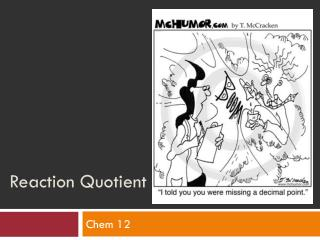DownloadDownload PresentationReaction Quotient

Reaction Quotient

Télécharger la présentationReaction Quotient

- - - - - - - - - - - - - - - - - - - - - - - - - - - E N D - - - - - - - - - - - - - - - - - - - - - - - - - - -
Presentation Transcript

1. Reaction Quotient Chem 12

2. More about equilibrium: • We’ve been looking at systems that reach equilibrium! • What if a reaction has not yet reached equilibrium? • How can you predict the direction in which the reaction must proceed to reach equilibrium? • We use the reaction quotient!

3. Reaction Quotient (Qc): • What does this look like? • ……. Kc or Keq • Used same way as Kc (or Keq) but uses concentrations not at equilibrium

4. The Relationship Between the Equilibrium Constant: • Qc > Kc: Reaction move right to left (from products to reactants) • Qc < Kc: Reaction move left to right (from reactants to products) • What if Qc = Kc ? • Then system is at equilibrium!

5. Think mathematically….

6. Example: • Calculate the Qc for the following reaction if these concentrations are present: [N2] = 0.10 M, [H2] = 0.30 M and [NH3] = 0.20 M. • Is the system at equilibrium if Kc of the reaction is 0.40? N2 + 3H2 < -- >2NH3

7. Example cont’d… N2 + 3H2 < -- >2NH3 1. Calculate Qc: Qc = [NH3]2= [0.20]2_______ [N2][H2]3 [0.10][0.30]3 Qc = 14.8 2. Compare Qc to Kc: 14.8 > 0.4 Qc > Kc (reaction shifts right to left (P to R)

8. Try it: • Page 519 # 30, 31